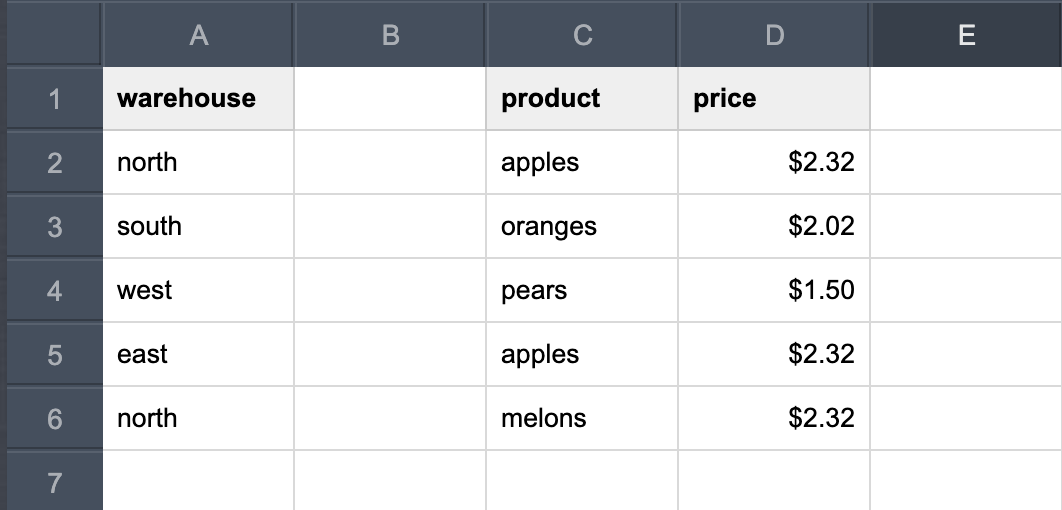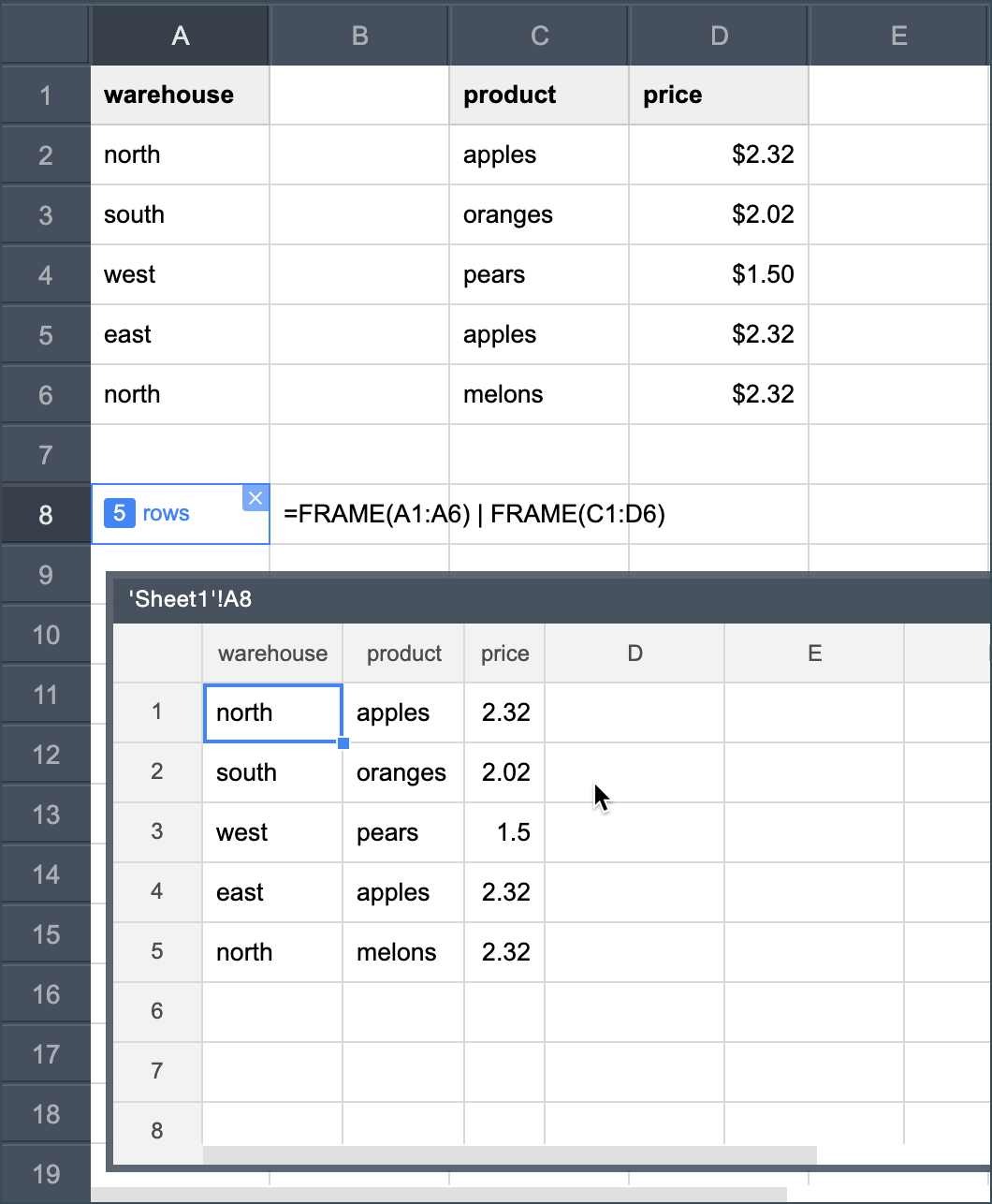# Combine frames

To combine two or more frames, use the pipe symbol "|" between them.

Let's take the following spreadsheet data as an example:To combine the above data into a single frame, use the following formula:

=FRAME(A1:A6) | FRAME(C1:D6)The resulting frame is shown in the figure below:Using the pipe symbol "|" to combine frames is not to be confused with using the pipe operator described here.

Contents